# C++ Program to Calculate Power Using Recursion

In this tutorial you will learn about the C++ Program to Calculate Power Using Recursion and its application with practical example.

## C++ program to Calculate Power Using Recursion

In this tutorial, we will learn to create a C++ program that will Calculate Power Using Recursion using C++ programming.

## Prerequisites

Before starting with this tutorial we assume that you are best aware of the following C++ programming topics:

• Operators in C++ Programming.
• Basic Input and Output function in C++ Programming.
• Basic C++ programming.
• Conditional Statements in C++ programming.
• Arithmetic operations in C++ Programming.

## What is Power?

The power of any number is shown as an exponent value of any number. This value shows exactly how many times the number is going to multiply by itself.

## Program to Calculate Power:-

In this tutorial, we will create a program that will take the base number and exponential number in input from the user and will calculate the answer using the recursion program.

The recursion is a program that repeats its execution again and again till a condition is satisfied.

Below is the program to Calculate Power Using Recursion.

## Output:-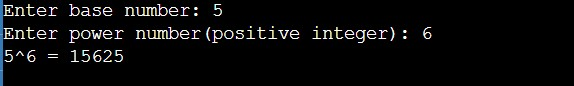In the above program, we have first initialized the required variable.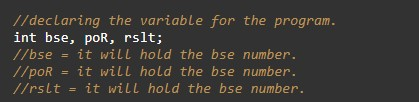• bse = it will hold the integer value.
• poR = it will hold the integer value.
• rslt = it will hold the integer value.
• j = it will hold the integer value.
• k = it will hold the integer value.
Taking input from the user the base and the power.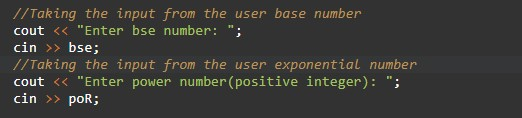Calling the function.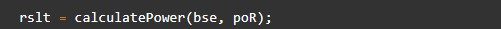Body of the function.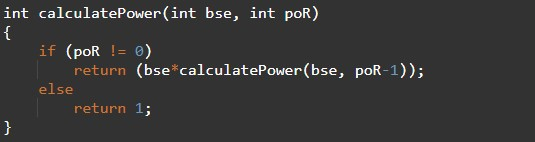Program Logic Code to print.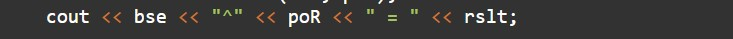In this tutorial we have learn about the C++ Program to Calculate Power Using Recursion and its application with practical example. I hope you will like this tutorial.